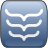## Ferrari's quartic equation solver in PHP

### Description

This library contains two robust functions to solve depressed and general cases of quartic equations with the use of Lodovico Ferrari's algorithm. The library depends on the lightweight suopte Complex class, which is also included in the repository.

### Introduction

Working on the StellaSphaera project I bumped into a geometric problem which involved the solution of a quartic equation. After long hesitation I decided to take a deep breath and dive into the world of complex numbers and quartic equations. Because I couldn't find any complex number class except the compliant and very readable but pretty cumbersome PEAR library - I found it better to create my own (suopte Complex). Then I started my journey in Lodovico Ferrari's labyrinth. Eventually with the guidance of the great Renaissance mathematician I easily got out of the maze.

### Reference

teFerrari
Class representing Lodovico Ferrari's quartic equation solver algorithm.
Methods:
depressedFerrari(float alpha, float beta, float gamma)
This function returns the four possible solutions of a depressed quartic equation (x4 + αx2 + βx + γ = 0).
Arguments
float alpha : Coefficient of the squared term in the above equation.
float beta : Coefficient of the first degree term in the above equation.
float gamma : Constant term in the above equation.
Return value
array Returns an array of four teComplex objects, containing the solutions.
generalFerrari(float A, float B, float C, float D, float E)
This function returns the four possible solutions of a general quartic equation (Ax4 + Bx3 + Cx2 + Dx + E = 0).
Arguments
float A : Coefficient of the quartic term in the above equation.
float B : Coefficient of the cubic term in the above equation.
float C : Coefficient of the quadratic term in the above equation.
float D : Coefficient of the first degree term in the above equation.
float E : Constant term in the above equation.
Return value
array Returns an array of four teComplex objects, containing the solutions.

Coming soon!

### Sources

 Repo: Ferrari PHP Date: 2013.05.13. License: MIT License Language: PHP Author: Bence Ágg
Reference content: